# step

Step response plot of dynamic system; step response data

## Syntax

``step(sys)``
``step(sys,tFinal)``
``step(sys,t)``
``step(sys,t,p)``
``step(sys1,sys2,...,sysN,___)``
``step(sys1,LineSpec1,...,sysN,LineSpecN,___)``
``step(___,config)``
``y = step(sys,t)``
``y = step(sys,t,p)``
``[y,tOut] = step(sys)``
``[y,tOut] = step(sys,tFinal)``
``[y,t,x] = step(sys)``
``[y,t,x,ysd] = step(sys)``
``[y,t,x,ysd,pOut] = step(sys,t,p)``
``[___] = step(___,config)``

## Description

### Step Response Plots

example

````step(sys)` plots the response of a dynamic system model to a step input of unit amplitude. The model `sys` can be continuous- or discrete-time, and SISO or MIMO. For MIMO systems, the plot displays the step responses for each I/O channel. `step` automatically determines the time steps and duration of the simulation based on the system dynamics.```

example

````step(sys,tFinal)` simulates the step response from `t = 0` to the final time ```t = tFinal```. The function uses system dynamics to determine the intervening time steps.```

example

````step(sys,t)` plots the step response at the times that you specify in the vector `t`. ```

example

````step(sys,t,p)` plots the step response for LPV model with parameter trajectory `p`.```

example

````step(sys1,sys2,...,sysN,___)` plots the step response of multiple dynamic systems on the same plot. All systems must have the same number of inputs and outputs. You can use multiple dynamic systems with any of the previous input-argument combinations.```

example

````step(sys1,LineSpec1,...,sysN,LineSpecN,___)` specifies a color, line style, and marker for each system in the plot. You can use `LineSpec` with any of the previous input-argument combinations. When you need additional plot customization options, use `stepplot` instead.```

example

````step(___,config)` specifies additional options for computing the step response, such as the step amplitude or input offset. Use `RespConfig` to create the option set `config`. You can use `config` with any of the previous input-argument and output-argument combinations. ```

### Step Response Data

example

````y = step(sys,t)` returns the step response of a dynamic system model `sys` at the times specified in the vector `t`. This syntax does not draw a plot.```
````y = step(sys,t,p)` also specifies the parameter trajectory `p` for LPV models.```
````[y,tOut] = step(sys)` also returns a vector of times `tOut` corresponding to the responses in `y`. If you do not provide an input vector `t` of times, `step` chooses the length and time step of `tOut` based on the system dynamics. ```
````[y,tOut] = step(sys,tFinal)` computes the step response up to the end time `tFinal`. `step` chooses the time step of `tOut` based on the system dynamics. ```
````[y,t,x] = step(sys)` also returns the state trajectories `x`, when `sys` is a state-space model such as an `ss` or `idss` model.```

example

````[y,t,x,ysd] = step(sys)` also computes the standard deviation `ysd` of the step response `y`, when `sys` is an identified model such as an `idss`, `idtf`, or `idnlarx` model.```
````[y,t,x,ysd,pOut] = step(sys,t,p)` also returns parameter trajectories `pOut`, when `sys` is an `lpvss` model.```

example

````[___] = step(___,config)` specifies additional options for computing the step response, such as the step amplitude or input offset. Use `RespConfig` to create the option set `config`. You can use `config` with any of the previous input-argument and output-argument combinations. ```

## Examples

collapse all

Plot the step response of a continuous-time system represented by the following transfer function.

`$\mathrm{sys}\left(\mathit{s}\right)=\frac{4}{{\mathit{s}}^{2}+2\mathit{s}+10}.$`

For this example, create a `tf` model that represents the transfer function. You can similarly plot the step response of other dynamic system model types, such as zero-pole gain (`zpk`) or state-space (`ss`) models.

`sys = tf(4,[1 2 10]);`

Plot the step response.

`step(sys)`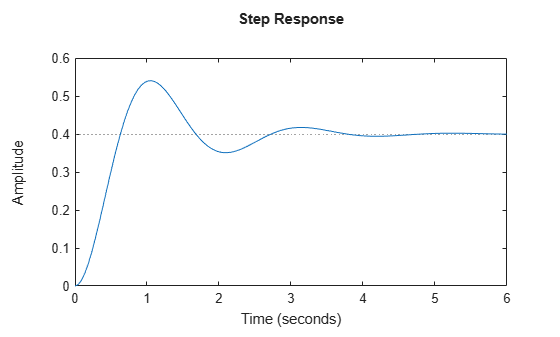The `step` plot automatically includes a dotted horizontal line indicating the steady-state response. In a MATLAB® figure window, you can right-click on the plot to view other step-response characteristics such as peak response and settling time. For more information about these characteristics, see `stepinfo`.

Plot the step response of a discrete-time system. The system has a sample time of 0.2 s and is represented by the following state-space matrices.

```A = [1.6 -0.7; 1 0]; B = [0.5; 0]; C = [0.1 0.1]; D = 0;```

Create the state-space model and plot its step response.

```sys = ss(A,B,C,D,0.2); step(sys)```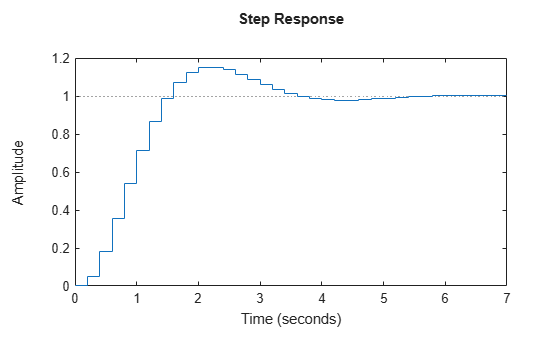The step response reflects the discretization of the model, showing the response computed every 0.2 seconds.

Examine the step response of the following transfer function.

`sys = zpk(-1,[-0.2+3j,-0.2-3j],1) * tf([1 1],[1 0.05]) `
```sys = (s+1)^2 ---------------------------- (s+0.05) (s^2 + 0.4s + 9.04) Continuous-time zero/pole/gain model. ```
`step(sys)`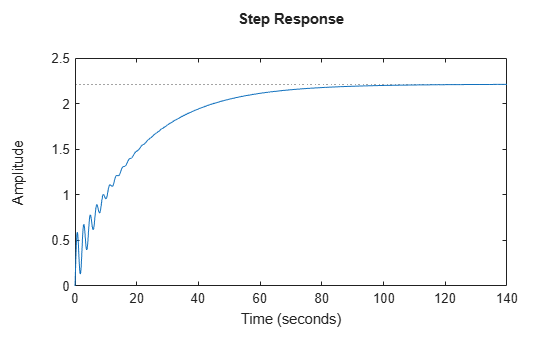By default, `step` chooses an end time that shows the steady state that the response is trending toward. This system has fast transients, however, which are obscured on this time scale. To get a closer look at the transient response, limit the step plot to `t` = 15 s.

`step(sys,15)`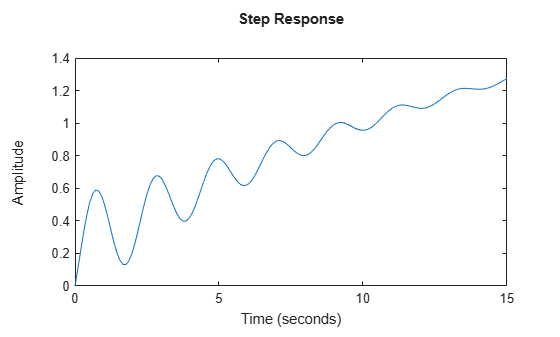Alternatively, you can specify the exact times at which you want to examine the step response, provided they are separated by a constant interval. For instance, examine the response from the end of the transient until the system reaches steady state.

```t = 20:0.2:120; step(sys,t)```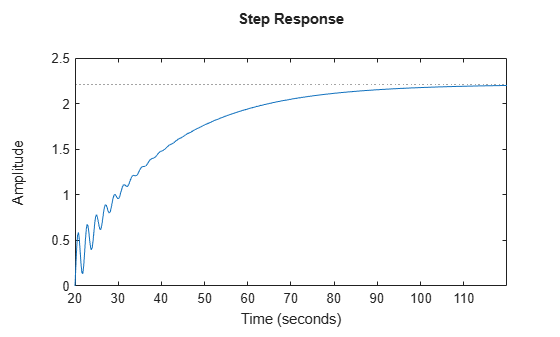Even though this plot begins at `t` = 20, `step` always applies the step input at `t` = 0.

Consider the following second-order state-space model:

`$\begin{array}{l}\left[\begin{array}{c}{\underset{}{\overset{˙}{x}}}_{1}\\ {\underset{}{\overset{˙}{x}}}_{2}\end{array}\right]=\left[\begin{array}{cc}-0.5572& -0.7814\\ 0.7814& 0\end{array}\right]\left[\begin{array}{c}{x}_{1}\\ {x}_{2}\end{array}\right]+\left[\begin{array}{cc}1& -1\\ 0& 2\end{array}\right]\left[\begin{array}{c}{u}_{1}\\ {u}_{2}\end{array}\right]\\ y=\left[\begin{array}{cc}1.9691& 6.4493\end{array}\right]\left[\begin{array}{c}{x}_{1}\\ {x}_{2}\end{array}\right]\end{array}$`

```A = [-0.5572,-0.7814;0.7814,0]; B = [1,-1;0,2]; C = [1.9691,6.4493]; sys = ss(A,B,C,0);```

This model has two inputs and one output, so it has two channels: from the first input to the output, and from the second input to the output. Each channel has its own step response.

When you use `step`, it computes the responses of all channels.

`step(sys)`The left plot shows the step response of the first input channel, and the right plot shows the step response of the second input channel. Whenever you use `step` to plot the responses of a MIMO model, it generates an array of plots representing all the I/O channels of the model. For instance, create a random state-space model with five states, three inputs, and two outputs, and plot its step response.

```sys = rss(5,2,3); step(sys)```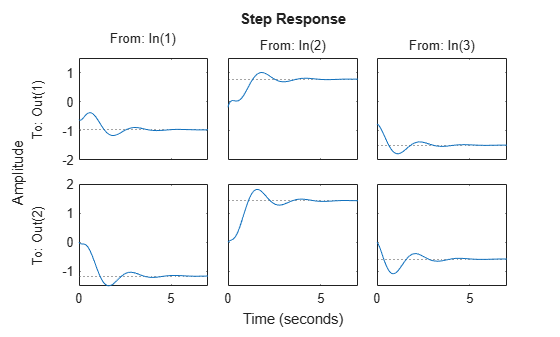In a MATLAB figure window, you can restrict the plot to a subset of channels by right-clicking on the plot and selecting I/O Selector.

`step` allows you to plot the responses of multiple dynamic systems on the same axis. For instance, compare the closed-loop response of a system with a PI controller and a PID controller. Create a transfer function of the system and tune the controllers.

```H = tf(4,[1 2 10]); C1 = pidtune(H,'PI'); C2 = pidtune(H,'PID');```

Form the closed-loop systems and plot their step responses.

```sys1 = feedback(H*C1,1); sys2 = feedback(H*C2,1); step(sys1,sys2) legend('PI','PID','Location','SouthEast')```By default, `step` chooses distinct colors for each system that you plot. You can specify colors and line styles using the `LineSpec` input argument.

``` step(sys1,'r--',sys2,'b') legend('PI','PID','Location','SouthEast')```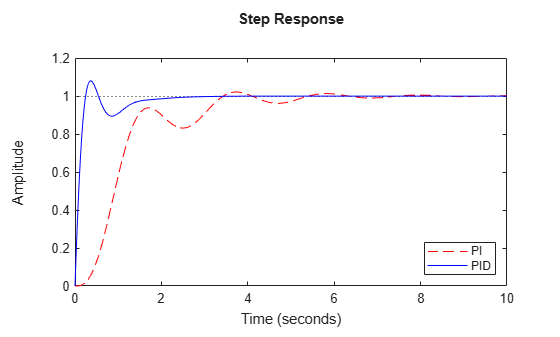The first `LineSpec` `'r--'` specifies a dashed red line for the response with the PI controller. The second `LineSpec` `'b'` specifies a solid blue line for the response with the PID controller. The legend reflects the specified colors and linestyles. For more plot customization options, use `stepplot`.

The example Compare Responses of Multiple Systems shows how to plot responses of several individual systems on a single axis. When you have multiple dynamic systems arranged in a model array, `step` plots all their responses at once.

Create a model array. For this example, use a one-dimensional array of second-order transfer functions having different natural frequencies. First, preallocate memory for the model array. The following command creates a 1-by-5 row of zero-gain SISO transfer functions. The first two dimensions represent the model outputs and inputs. The remaining dimensions are the array dimensions.

` sys = tf(zeros(1,1,1,5));`

Populate the array.

```w0 = 1.5:1:5.5; % natural frequencies zeta = 0.5; % damping constant for i = 1:length(w0) sys(:,:,1,i) = tf(w0(i)^2,[1 2*zeta*w0(i) w0(i)^2]); end```

(For more information about model arrays and how to create them, see Model Arrays.) Plot the step responses of all models in the array.

`step(sys)`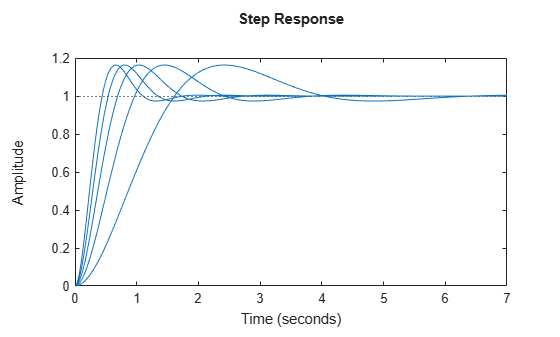`step` uses the same linestyle for the responses of all entries in the array. One way to distinguish among entries is to use the `SamplingGrid` property of dynamic system models to associate each entry in the array with the corresponding `w0` value.

`sys.SamplingGrid = struct('frequency',w0);`

Now, when you plot the responses in a MATLAB figure window, you can click a trace to see which frequency value it corresponds to.

When you give it an output argument, `step` returns an array of response data. For a SISO system, the response data is returned as a column vector of length equal to the number of time points at which the response is sampled. You can provide the vector t of time points, or allow `step` to select time points for you based on system dynamics. For instance, extract the step response of a SISO system at 101 time points between t = 0 and t = 5 s.

```sys = tf(4,[1 2 10]); t = 0:0.05:5; y = step(sys,t); size(y)```
```ans = 1×2 101 1 ```

For a MIMO system, the response data is returned in an array of dimensions N-by-Ny-by-Nu, where Ny and Nu are the number of outputs and inputs of the dynamic system. For instance, consider the following state-space model, representing a two-input, one-output system.

```A = [-0.5572,-0.7814;0.7814,0]; B = [1,-1;0,2]; C = [1.9691,6.4493]; sys = ss(A,B,C,0);```

Extract the step response of this system at 200 time points between t = 0 and t = 20 s.

```t = linspace(0,20,200); y = step(sys,t); size(y)```
```ans = 1×3 200 1 2 ```

`y(:,i,j)` is a column vector containing the step response from the jth input to the ith output at the times t. For instance, extract the step response from the second input to the output.

```y12 = y(:,1,2); plot(t,y12)```Create a feedback loop with delay and plot its step response.

```s = tf('s'); G = exp(-s) * (0.8*s^2+s+2)/(s^2+s); sys = feedback(ss(G),1); step(sys)```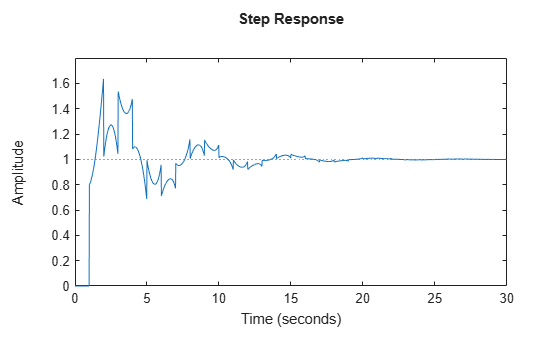The system step response displayed is chaotic. The step response of systems with internal delays may exhibit odd behavior, such as recurring jumps. Such behavior is a feature of the system and not software anomalies.

By default, `step` applies an input signal that changes from 0 to 1 at t = 0. To customize the amplitude and offset, use `RespConfig`. For instance, compute the response of a SISO state-space model to a signal that changes from 1 to –1 to at t = 0.

```A = [1.6 -0.7; 1 0]; B = [0.5; 0]; C = [0.1 0.1]; D = 0; sys = ss(A,B,C,D,0.2); opt = RespConfig; opt.InputOffset = 1; opt.Amplitude = -2; step(sys,opt)```For responses to arbitrary input signals, use `lsim`.

Compare the step response of a parametric identified model to a non-parametric (empirical) model. Also view their 3 $\sigma$ confidence regions.

`load iddata1 z1`

Estimate a parametric model.

`sys1 = ssest(z1,4);`

Estimate a non-parametric model.

`sys2 = impulseest(z1);`

Plot the step responses for comparison.

```t = (0:0.1:10)'; [y1, ~, ~, ysd1] = step(sys1,t); [y2, ~, ~, ysd2] = step(sys2,t); plot(t, y1, 'b', t, y1+3*ysd1, 'b:', t, y1-3*ysd1, 'b:') hold on plot(t, y2, 'g', t, y2+3*ysd2, 'g:', t, y2-3*ysd2, 'g:')```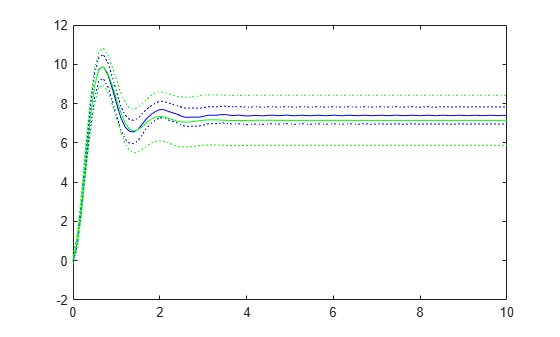Compute the step response of an identified time-series model.

A time-series model, also called a signal model, is one without measured input signals. The step plot of this model uses its (unmeasured) noise channel as the input channel to which the step signal is applied.

`load iddata9;`

Estimate a time-series model.

`sys = ar(z9, 4);`

`sys` is a model of the form `A y(t) = e(t)` , where `e(t)` represents the noise channel. For computation of step response, `e(t)` is treated as an input channel, and is named `e@y1`.

Plot the step response.

`step(sys)`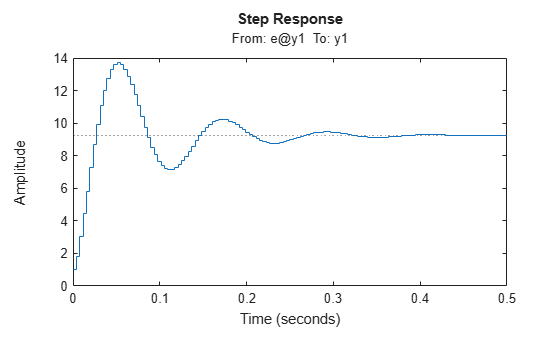Validate the linearization of a nonlinear ARX model by comparing the small amplitude step responses of the linear and nonlinear models.

`load iddata2 z2;`

Estimate a nonlinear ARX model.

```nlsys = nlarx(z2,[4 3 10],idTreePartition,'custom',... {'sin(y1(t-2)*u1(t))+y1(t-2)*u1(t)+u1(t).*u1(t-13)',... 'y1(t-5)*y1(t-5)*y1(t-1)'},'nlr',[1:5, 7 9]);```

Determine an equilibrium operating point for `nlsys` corresponding to a steady-state input value of 1.

```u0 = 1; [X,~,r] = findop(nlsys, 'steady', 1); y0 = r.SignalLevels.Output;```

Obtain a linear approximation of `nlsys` at this operating point.

`sys = linearize(nlsys,u0,X);`

Validate the usefulness of `sys` by comparing its small-amplitude step response to that of `nlsys`.

The nonlinear system `nlsys` is operating at an equilibrium level dictated by `(u0,y0)`. Introduce a step perturbation of size 0.1 about this steady-state and compute the corresponding response.

```opt = RespConfig; opt.InputOffset = u0; opt.Amplitude = 0.1; t = (0:0.1:10)'; ynl = step(nlsys, t, opt);```

The linear system `sys` expresses the relationship between the perturbations in input to the corresponding perturbation in output. It is unaware of the nonlinear system's equilibrium values.

Plot the step response of the linear system.

```opt = RespConfig; opt.Amplitude = 0.1; yl = step(sys, t, opt);```

Add the steady-state offset, `y0` , to the response of the linear system and plot the responses.

```plot(t, ynl, t, yl+y0) legend('Nonlinear', 'Linear with offset')```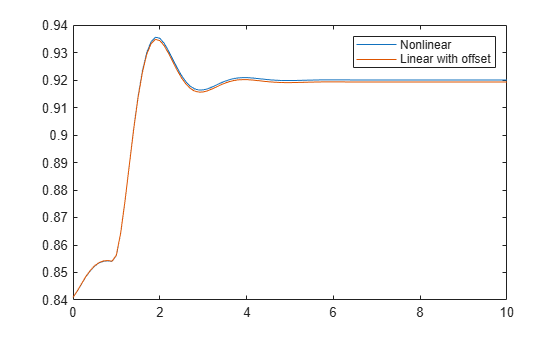This example shows how to simulate the step response of an LPV model. This example simulates the closed-loop step response of a levitating ball model defined in `fcnMaglev.m` to a disturbance $\mathit{du}$.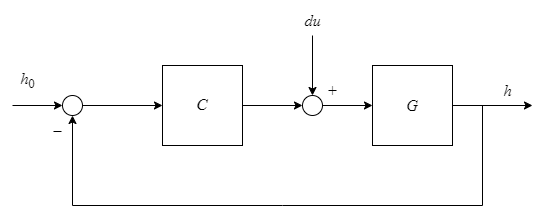Create the model and discretize it.

```hmin = 0.05; hmax = 0.25; h0 = (hmin+hmax)/2; Ts = 0.01; Glpv = lpvss("h",@fcnMaglev,0,0,h0); Glpvd = c2d(Glpv,Ts,'tustin'); ```

Sample the LPV model for three height values and tune a PID controller.

```hpid = linspace(hmin,hmax,3); [Ga,Goffset] = sample(Glpvd,[],hpid); wc = 50; Ka = pidtune(Ga,'pidf',wc); Ka.Tf = 0.01;```

Create the gain-scheduled PID controller.

```Ka.SamplingGrid = struct('h',hpid); Koffset = struct('y',{Goffset.u}); Clpv = ssInterpolant(ss(Ka),Koffset);```

Create the closed-loop model.

```CL = feedback(Glpvd*[1,Clpv],1,2,1); CL.InputName = {'du';'href'}; CL.OutputName = 'h';```

Get steady-state current for $\mathit{h}$ = ${\mathit{h}}_{0}$ to size the disturbance

`[~,~,~,~,~,~,~,u0] = Glpv.DataFunction(0,h0);`

Step response to input disturbance and step change in reference. Set the input offset $\mathit{du}$ = 0 and $\mathit{h}$ = ${\mathit{h}}_{0}$ to specify the starting steady-state condition.

```t = 0:Ts:2; pFcn = @(k,x,u) x(1); Config = RespConfig('InputOffset',[0;h0],'Amplitude',0.2*[u0;h0]*Ts,'Delay',0.5,'InitialParameter',h0); step(CL,t,pFcn,Config) title('Step Disturbance in Current and Step Change in Height')```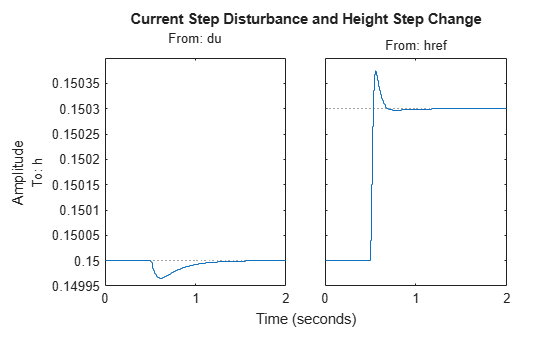## Input Arguments

collapse all

Dynamic system, specified as a SISO or MIMO dynamic system model or array of dynamic system models. Dynamic systems that you can use include:

• Continuous-time or discrete-time numeric LTI models, such as `tf`, `zpk`, or `ss` models.

• Generalized or uncertain LTI models such as `genss` or `uss` models. (Using uncertain models requires Robust Control Toolbox™ software.)

• For tunable control design blocks, the function evaluates the model at its current value for both plotting and returning response data.

• For uncertain control design blocks, the function plots the nominal value and random samples of the model. When you use output arguments, the function returns response data for the nominal model only.

• Sparse state-space models such as `sparss` and `mechss` models.

• Identified LTI models, such as `idtf`, `idss`, or `idproc` models. For such models, the function can also plot confidence intervals and return standard deviations of the frequency response. See Step Responses of Identified Models with Confidence Regions. (Using identified models requires System Identification Toolbox™ software.)

• Linear time-varying (`ltvss`) and linear parameter-varying (`lpvss`) models.

`step` does not support frequency-response data models such as `frd`, `genfrd`, or `idfrd` models.

If `sys` is an array of models, the function plots the responses of all models in the array on the same axes. See Step Response of Systems in a Model Array.

End time for the step response, specified as a positive scalar value. `step` simulates the step response from `t = 0` to `t = ``tFinal`.

• For continuous-time systems, the function determines the step size and number of points automatically from system dynamics. Express `tFinal` in the system time units, specified in the `TimeUnit` property of `sys`.

• For discrete-time systems, the function uses the sample time of `sys` as the step size. Express `tFinal` in the system time units, specified in the `TimeUnit` property of `sys`.

• For discrete-time systems with unspecified sample time (```Ts = -1```), `step` interprets `tFinal` as the number of sampling periods to simulate.

Time vector at which to compute the step response, specified as a vector of positive scalar values. Express `t` in the system time units, specified in the `TimeUnit` property of `sys`.

• For continuous-time models, specify `t` in the form `T0:dt:Tf`. To obtain the response at each time step, the function uses `dt` as the sample time of a discrete approximation to the continuous system (see Algorithms). The simulation starts at `T0` and ends at `Tf`.

• For discrete-time models, specify `t` in the form `T0:Ts:Tf`, where `Ts` is the sample time of `sys`.

Line style, marker, and color, specified as a string or vector of one, two, or three characters. The characters can appear in any order. You do not need to specify all three characteristics (line style, marker, and color). For example, if you omit the line style and specify the marker, then the plot shows only the marker and no line. For more information about configuring this argument, see the `LineSpec` input argument of the `plot` function.

Example: `'r--'` specifies a red dashed line

Example: `'*b'` specifies blue asterisk markers

Example: `'y'` specifies a yellow line

Parameter trajectory of the LPV model, specified as a matrix or a function handle.

• For exogenous or explicit trajectories, specify `p` as a matrix with dimensions N-by-Np, where N is the number of time samples and Np is the number of parameters.

Thus, the row vector `p(i,:)` contains the parameter values at the ith time step.

• For endogenous or implicit trajectories, specify `p` as a function handle of the form p = F(t,x,u) in continuous time and p = F(k,x,u) in discrete time that gives parameters as a function of time t or time sample k, state x, and input u.

This option is useful when you want to simulate quasi-LPV models. For an example, see Step Response of LPV Model.

Configuration of the applied signal, specified as a `RespConfig` option set. By default, `step` applies an input that goes from 0 to 1 at time `t = 0`. Use this input argument to change the configuration of the step input. See Response to Custom Step Input for an example.

For `lpvss` and `ltvss` models with offsets (x0(t),u0(t)), you can use `RespConfig` to define the input relative to u0(t,p) and initialize the simulation with the state x0(t,p).

## Output Arguments

collapse all

Step response data, returned as an array.

• For SISO systems, `y` is a column vector of the same length as `t` (if provided) or `tOut` (if you do not provide `t`).

• For single-input, multi-output systems, `y` is a matrix with as many rows as there are time samples and as many columns as there are outputs. Thus, the jth column of `y`, or `y(:,j)`, contains the step response of from the input to the jth output.

• For MIMO systems, the step responses of each input channel are stacked up along the third dimension of `y`. The dimensions of `y` are then N-by-Ny-by-Nu, where:

• N is the number of time samples.

• Ny is the number of system outputs.

• Nu is the number of system inputs.

Thus, `y(:,i,j)` is a column vector containing the step response from the jth input to the ith output at the times specified in `t` or `tOut`.

Times at which step response is computed, returned as a vector. When you do not provide a specific vector `t` of times, `step` chooses this time vector based on the system dynamics. The times are expressed in the time units of `sys`.

State trajectories, returned as an array. When `sys` is a state-space model, `x` contains the evolution of the states of `sys` at each time in `t` or `tOut`. The dimensions of `x` are N-by-Nx-by-Nu, where:

• N is the number of time samples.

• Nx is the number of states.

• Nu is the number of system inputs.

Thus, the evolution of the states in response to a step injected at the kth input is given by the array `x(:,:,k)`. The row vector `x(i,:,k)` contains the state values at the ith time step.

Standard deviation of the step response of an identified model, returned as an array of the same dimensions as `y`. If `sys` does not contain parameter covariance information, then `ysd` is empty.

Parameter trajectories, returned as an array. When `sys` is a linear-parameter varying model, `pOut` contains the evolution of the parameters of `sys` at each time in `t` or `tOut`. The dimensions of `pOut` are N-by-Np-by-Nu, where:

• N is the number of time samples.

• Np is the number of parameters.

• Nu is the number of system inputs.

Thus, the evolution of the parameters in response to a step injected at the kth input is given by the array `pOut(:,:,k)`. The row vector `pOut(i,:,k)` contains the parameter values at the ith time step.

## Algorithms

To obtain samples of continuous-time models without internal delays, `step` converts such models to state-space models and discretizes them using a zero-order hold on the inputs. `step` chooses the sampling time for this discretization automatically based on the system dynamics, except when you supply the input time vector `t` in the form `t = T0:dt:Tf`. In that case, `step` uses `dt` as the sampling time. The resulting simulation time steps `tOut` are equisampled with spacing `dt`.

For systems with internal delays, Control System Toolbox™ software uses variable step solvers. As a result, the time steps `tOut` are not equisampled.

 L.F. Shampine and P. Gahinet, "Delay-differential-algebraic equations in control theory," Applied Numerical Mathematics, Vol. 56, Issues 3–4, pp. 574–588.